# Understanding the Face Recognition Algorithms

In today’s world, biometric sensors or devices used for security purposes. Biometric devices use because they provide more security and reliability. One of the techniques in biometric devices is Face recognition.

## What is face recognition?

Face recognition is used to identify the person from their image or video. Some features like eyes, lips, shape, etc. extract from the image or video source to identify the person’s identity. The face recognition system is used in biometric devices because of more security and easy to use. There are many algorithms are used in the face recognition system. Here we discuss a few of them.

## Face recognition algorithms

• Eigenfaces
• Fisherfaces
• Local Binary Pattern Histograms(LBPH):

### Eigenfaces:

Eigenfaces is a face recognition algorithm, which uses principal component analysis(PCA). PCA is a statistical approach that is used for dimensionality reduction. Eigenfaces reduce some less important features from the image and take only important and necessary features of the image. Eigenfaces reduce dimensionality with having important features of the image. When dimensionality reduced then quality and space occupied will also reduce because of losing information(less important).

First, we take an input image from the database. We need a large dataset for training for getting a more accurate result. After taking the image, classify the image using an image classifier. We can use a single layer neural networks in our classifier. In this classifier, we make a 2D image to vector (If image size is pxq then we make a vector of pqx1). Then we are doing the process of feature extraction.

Algorithm:

In Eigenfaces we are using PCA. From 2D image we get to 1D vector it called feature vector.

PCA performs as given below

Let P={P1,P2,…,Pn} is random vector.

1. Find mean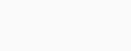2. Find Covariance Matrix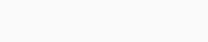3. Find eigenvector λi and eigenvalue bi of S : Sbi = λibi ,i=1,2,…,n
4. Sort all eigenvectors by their value and select the first k largest eigenvectors. So the new observed vector is given by

v = WT(P – m)

where W = {b1,b2,…,bk}

From using PCA we get eigenvector and dimensionality smaller that original dimensionality (pqx1) From classifier we get if the image is matching or not.

### Fisherfaces:

Fisherfaces is also a face recognition algorithm. In the Fisherfaces algorithm, we only find the feature that distinguishes one from the other for that we are using linear discriminant analysis(LDA). LDA is used for class separability and dimensionality reduction by a linear combination of features. Fisherfaces use features that distinguish one from others instead of using the common feature. Fisherfaces is more efficient when there is a large number of dataset of facial images with various expression.

Algorithm:

First, we take input images from the database. After taking the image to get features from the data we use a classifier to classify the image. In classifier, LDA is used for discretization.

Linear discriminant analysis(LDA) performed by the following steps:

There are x different classes:

P={P1, P2, …,Px}

Here, Pi is the sample.

Pi={p1, p2, …, pn}

We compute the scatter matrix using the following formulaTotal Mean: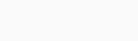Mean of all class from 1 to x: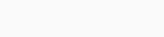Then we solve the eigenvalue problem to obtain linear discriminant:

(SW)-1SB

From eigenvector, we get a new feature space. From LDA we get different separate classes. With the help of LDA, we can get the feature space from that classifier to classify the image if it is matching or not.

Fisherfaces needs more storage size and more processing time for face recognition.

### Local Binary Pattern Histograms(LBPH):

LBPH widely used algorithm for face recognition. In addition, LBPH is a very simple and efficient approach to face recognition. Therefore LBPH uses Histograms.

In LBPH we compare every pixel of the image with its neighboring pixel. Center pixel is thresholding pixel and its value is a threshold value. The other neighboring pixel values are compared with the threshold value. If the value is more than a threshold then it is set to one(1) and if it is less threshold value then it is set to zero(0). This value is converted to binary by concate all the neighbor pixels. The pixels can concate in a clockwise direction or anticlockwise direction.

As shown in the below example we are concate pixels In clockwise direction and making it binary.First, we take an input image and detect face from it. Then applying LBPH to recognize the face.

We can find LBP by the following formula: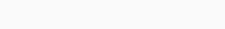Here,(xc,yc)   is a center pixel, tc is the intensity of center pixel and tp the intensity of the neighboring pixel

F(x)={1; x ≥ 0 ,

0 ; x < 0}

From getting value in binary we convert it into decimal. Then every pixel of image assigns a new value. From the new value of the image, we form a histogram. All the local histogram is concatenating to obtain a feature vector. This is Local Binary Pattern Histograms.

### Conclusion:

In conclusion, we learned the following algorithm for face recognition in this tutorial.

• Eigenfaces
• Fisherfaces
• Local binary pattern histogram(LBPH)

### One response to “Understanding the Face Recognition Algorithms”

1. AOUATIF says:

can i use lbph for recognise another object if it yes can you give me the source for i can do the same with my object can you give me any information hepful please?